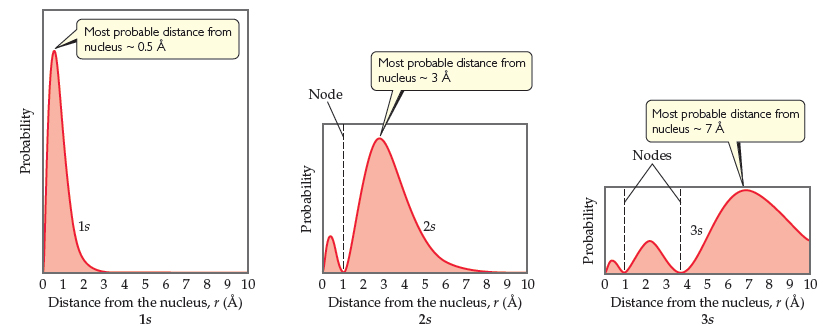# Problem: Radial probability distributions for the 1s , 2s , and 3s  orbitals of hydrogen. These plots show the probability of finding the electron as a function of distance from the nucleus. As n increases, the most likely distance at which to find the electron (the highest peak) moves farther from the nucleus.How many maxima would you expect to find in the radial probability function for the 4s  orbital of the hydrogen atom?

###### FREE Expert Solution

From the radial probability distribution plots, we can see that:

• principal quantum number, n → corresponds to n peaks (or maxima)
• 1s has 1 maxima, 2s has 2 maxima, 3s has 3 maxima

91% (134 ratings)###### Problem DetailsRadial probability distributions for the 1s , 2s , and 3s  orbitals of hydrogen. These plots show the probability of finding the electron as a function of distance from the nucleus. As n increases, the most likely distance at which to find the electron (the highest peak) moves farther from the nucleus.

How many maxima would you expect to find in the radial probability function for the 4s  orbital of the hydrogen atom?

Frequently Asked Questions

What scientific concept do you need to know in order to solve this problem?

Our tutors have indicated that to solve this problem you will need to apply the Quantum Numbers: Nodes concept. You can view video lessons to learn Quantum Numbers: Nodes. Or if you need more Quantum Numbers: Nodes practice, you can also practice Quantum Numbers: Nodes practice problems.

What professor is this problem relevant for?

Based on our data, we think this problem is relevant for Professor Tang's class at USF.